The code below implements some quadtree extensions, as discussed in another Dev.Mag tutorial about quadtrees (see Issue 27). The tutorial covers the following topics:

• what to consider in choosing whether to use a quadtree or not;
• how to handle discrete data; and
• some modifications to the basic algorithm.

## Handling Discrete Data

When it comes to discrete data, the “average” of a number of pixels doesn’t make sense. However, we can give it meaning and still use it to get good approximations of the original data, as illustrated in the images below.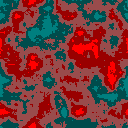The original grid of discrete data. The grid contains integers from 0 to 4. Every integer is mapped to a colour in this image. (If this grid represented a tile map, every integer would be mapped to a different tile).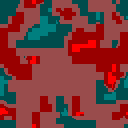Here we allowed floating point numbers in the quadtree. Results of queries into the quadtree are rounded before mapping them to colours.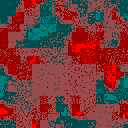Here we used the floating point part of queries to bias a randomly selected integer. For example, a result of 1.25 will result in a 75% chance of yielding 2.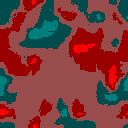Here we use a quadtree with interpolation. The result is rounded before it is mapped.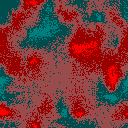Here we use a quadtree with interpolation, and use the floating point part of the number to bias a randomly selected tile, as above.

### Python Implementation1.Byder says:
2.admin says: